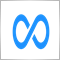# StrToDouble() and StringToDouble() adds unnecessary floating digits.275

`StringToDouble("80.198")`

Output: 80.19799999999999

Caught this by coincidence. I have tried all sorts of conversions, NormalizeDouble (string to double to string). so on and so forth. But still, it beats me to understand why this is happening.

Appreciate any help.

Thanks.27521

1. Floating-point has an infinite number of decimals, it's your not understanding floating-point and that some numbers can't be represented exactly. (like 1/10.)
Double-precision floating-point format - Wikipedia, the free encyclopedia

If you want to see the correct number of digits, convert it to a string with the correct/wanted accuracy.
question about decima of marketinfo() - MQL4 programming forum 2016.05.18

2. NormalizeDouble does not return a string.275

William Roeder:
1. Floating-point has an infinite number of decimals, it's your not understanding floating-point and that some numbers can't be represented exactly. (like 1/10.)
Double-precision floating-point format - Wikipedia, the free encyclopedia

If you want to see the correct number of digits, convert it to a string with the correct/wanted accuracy.
question about decima of marketinfo() - MQL4 programming forum 2016.05.18

2. NormalizeDouble does not return a string.

I am not calculating a new number; I am simply converting a string to a double. I do understand floating-point numbers and I had nightmares normalizing price with NormalizeDouble (in the beginning), but then read that it's not recommended, so switched to the MathRound() method.

I receive a STRING that I need to convert to a price I can work with. Question is simple, really: Is there a way (YES/NO), based on the code I inserted above, to convert "80.198" to an exact number representation of that string?Moderator
35007

Hussein Saade: Question is simple, really: Is there a way (YES/NO), based on the code I inserted above, to convert "80.198" to an exact number representation of that string?

Yes.

`double value=StringToDouble("80.198")`

Buy if you want to print it to 3 digits

`DoubleToString(value,3)`